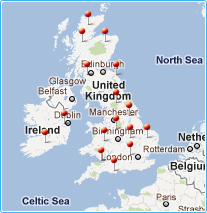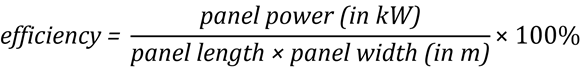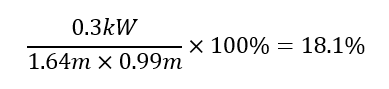#Tel.:+44 (0) 203 150 11 11

Fax:+44 (0) 203 150 12 12

Free UK delivery
• Items:
0
• Total:
£ 0.00

## Online shop

### Didn't find products you were looking for?

Tell us about them! We might have these products in stock, but not on the website, or may be we are planning to restock them soon.

### Testimonials:

This arrived so fast, it must have been beamed over! 5*++++

Peter (Shoreham-by-Sea, West Sussex, UK) - June 2012

#### We are a trusted supplier to thousands of UK clients## Articles

26.07.2019, 17:33

### How to Calculate Solar Panel Efficiency

When deciding on the components of your solar power system, you may be curious about the efficiency of the solar panels. This article explains exactly how this can be calculated, and everything else you need to know about solar panel efficiency.

The equation below can be used to calculate the approximate efficiency of a solar panel, as a percentage:Firstly, it is important to stress that efficiency of a solar panel is a matter of area, not power. A 10% efficient 100W panel and a 20% efficient 100W panel will both produce exactly the same amount of power. However, you should expect the 20% efficient solar panel to be half the total size of the 10% efficient panel.

To determine the efficiency of the panel, we need to compare the power that the sun provides to the panel to the electricity that is produced. If a panel was able to convert all of the light that falls on it into electricity, then that panel would be 100% efficient. Unfortunately, this level of efficiency is impossible.

The theoretical maximum efficiency of a solar cell made of ideal material is 33.7%. This is known as the Shockley-Quiesser limit, and is a consequence of the laws of physics and how solar cells absorb power. Multi-layered solar cells can exceed this limit in lab conditions, but are significantly harder to manufacture and much more expensive so are typically only used for satellites and other hi-tech systems where space is extremely limited.

For silicon solar cells (the kind in almost every panel you can buy) the theoretical limit is about 32% as silicon, though close, is not a perfect solar cell material. Mass market solar cells will always experience some power loss compared to this and can only achieve a maximum of up to 25% in ideal conditions. The efficiency of a panel as a whole will always be lower than its component cells due to the frame, reflective metal contacts, and gaps between the cells.

To begin comparing the sun’s power to the panel’s electricity output, we first need to know the sun’s power. At midday near the equator, just over 1kW (1000W) of sunlight reaches every square meter of the ground. Away from the equator and in different seasons, weather conditions and times of day this will be less, but the 1kW per square meter value is used when testing panels to give the power rating they are sold as. This part of the standard test conditions (STC) that the solar power industry uses, and all panels are rated in the same way.

Imagine a panel with an area of 1 square meter. If it produced 1kW of electricity at noon on the equator we could say that it was 100% efficient as it would receive 1kW of sunlight and turn that into the same amount of electricity. If a panel the same size instead produced 200W of power, then its efficiency is 200W/1000W x 100 = 20%. If we instead express this in kW then the equation becomes even simpler, as 0.2kW/1kW = 0.2 so the sun’s power can be ignored in the calculation. The ratio is multiplied by 100 to give an answer in percentage.

To perform this calculation for any solar panel that isn’t 1 square meter, we need to know the area of the panel. If a panel is half as big and produces the same power it is twice as efficient, and vice versa. The area can be easily calculated from the panel’s dimensions by multiplying the width by the length. Remember to convert the dimensions into meters first as areas do not convert in the same way as lengths (i.e. 1 square meter is not 1000 square millimetres, it is 1 million square millimetres).

Finally, to calculate the maximum efficiency of the solar panel we need to divide the ratio of panel power to sun power by the area of the panel in square meters, then times by 100 to get a percentage. Make sure the measurement units of all the values are correct or you will end up with very strange results.

Take, for example, our 300W rigid frame panel with monocrystalline silicon cells. Its power is 300W or 0.3kW, it is 1.64m long and 0.99m wide. The efficiency is therefore:This is the approximate efficiency of the panel as a whole, so as mentioned above we would expect it to be lower than the efficiency of the cells because of the frame and gaps between the solar cells (increasing the area) and normal losses as the electricity travels through the panel and wires. For a higher efficiency panel of the same area, the power would be higher than 300W. For a higher efficiency panel of the same power, the area would be smaller.